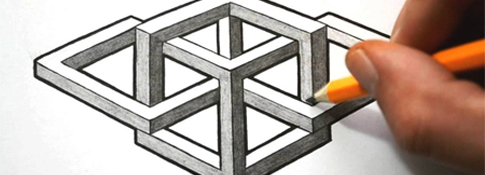##### Geometric Shapes #3Published: 3.22.2021
Level 1   |   Time: 1:10
Accent: New Zealand
Source: Listen In English

A simple drawing of geometric shapes including squares, circles, rectangles, triangles, vertical lines, horizonatal lines and diagonal lines.###Directions

1. REVIEW the vocabulary.
2. LISTEN to the audio above.
3. DRAW the picture (on a piece of paper).

###Vocabulary

If you don't know any of the words below, check the Illustrated Dictionary.

• a circle
• a square
• a triangle
• a star
• a line
• horizontal
• vertical

###Grammar (articles)

Directions: 1) Choose the determiner that you think best fits the sentence. 2) Listen again and check your answers.

• This is (a) picture of four figures – (a) square, (a) circle, (a) star, and (a) triangle.
• In (the) top left corner of (the) page, there is (a) medium-sized square.
• In (the) middle of (the) square, there is (a) small circle.
• In (the) top right corner of (the) page, there is (a) medium-sized circle.
• In (the) middle of (the) circle, there is (a) vertical line.
• In (the) bottom left corner of (the) page, there is (a) five-pointed star.
• Above (the) star, there is (a) small circle.
• In (the) bottom right corner of (the) page, there is (a) medium-sized triangle.
• Below (the) triangle, there is (a) horizontal line.

###Script

This is a picture of four figures – a square, a circle, a star, and a triangle.

In the top left corner of the page, there is a medium-sized square.

In the middle of the square, there is a small circle.

In the top right corner of the page, there is a medium-sized circle.

In the middle of the circle, there is a vertical line.

In the bottom left corner of the page, there is a five-pointed star.

Above the star, there is a small circle.

In the bottom right corner of the page, there is a medium-sized triangle.

Below the triangle, there is a horizontal line.